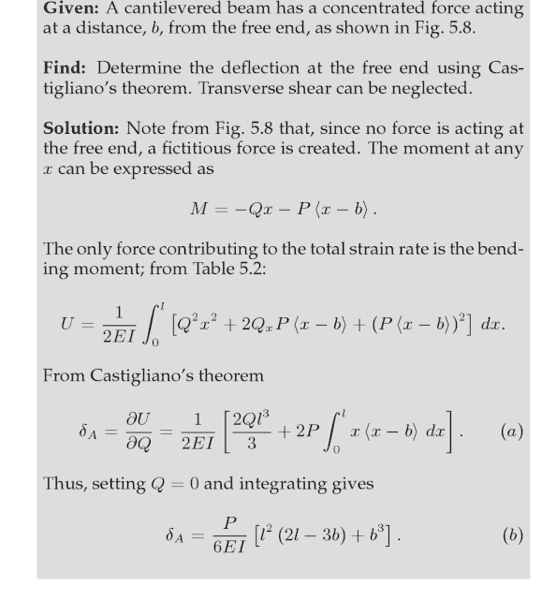# Castigaliano's Theorem for cantilever beams

## Homework Statement

Hi I am trying to derive an expression for the deflection in a cantilever beam using castigliano's theorem.
I have found and attached an example of the solution. I understand the most of what is going on in the attached solution but I don't know where the b^3 came from in the line (b)see attached[/B]

## The Attempt at a Solution

When I perform the integration from line (a) i get
L^2/6(2L-3b). Can anybody explain where the b^3 in line (b) comes from[/B]

#### Attachments

haruspex
Homework Helper
Gold Member
2020 Award
When I perform the integration from line (a) i get
L^2/6(2L-3b)
Remember what the <x-b> notation means. How will you integrate wrt x over 0 to l?

Are the angular brackets in <a-b> something to do with a singularity function, that is only turned on for certain cases.

haruspex
Homework Helper
Gold Member
2020 Award
Are the angular brackets in <a-b> something to do with a singularity function, that is only turned on for certain cases.
They mean (in the context of moments in beams etc.) max{a-b, 0}.

for max{x-b, 0}. I think x-b was chosen as the max in the attached example. so does this mean that <x-b>
can now be change to (x-b) ?

haruspex
Homework Helper
Gold Member
2020 Award
for max{x-b, 0}. I think x-b was chosen as the max in the attached example. so does this mean that <x-b>
can now be change to (x-b) ?
No.
x is the variable of integration and takes values from 0 to l. Draw a graph of <x-b> over that range. How are you going to integrate it?

I am having difficulty drawing the graph of <x - b> because I dont have a value for b yet. can you give me a further hint please.

haruspex
Homework Helper
Gold Member
2020 Award
I am having difficulty drawing the graph of <x - b> because I dont have a value for b yet. can you give me a further hint please.
I just meant a schematic graph, it does not have to be exact. x has some range. b is a point within that range.

you also mentioned about integrating from 0 to L. This would give me ((L^2)/6)*(2L-3B)) - ((0^2)/6)*(2(0)-3B)). is this correct ?

haruspex
Homework Helper
Gold Member
2020 Award
you also mentioned about integrating from 0 to L. This would give me ((L^2)/6)*(2L-3B)) - ((0^2)/6)*(2(0)-3B)). is this correct ?
No. You cannot integrate until you have figured out what to do with the <x-b>.
Have you tried to sketch a graph of <x-b> over the range 0 to L? Remember that 0<b<L. What does it look like?

I have plotted two charts with different values for b. One chart plots b at 0.1. the other chart plots b at 0.9.
I see in both cases that some of the <x-B> values are below the x-axis and some of the <x-b> are above the x-axis.

haruspex
Homework Helper
Gold Member
2020 Award
some of the <x-B> values are below the x-axis
By definition (see post #4) it is never below the x axis.

ok. So i guess I have to integrate between the limits of <x-b> greater than 0 and less that L. but how do i know if x-b is greater than 0 if I don't have a value for b.

haruspex
Homework Helper
Gold Member
2020 Award
ok. So i guess I have to integrate between the limits of <x-b> greater than 0 and less that L. but how do i know if x-b is greater than 0 if I don't have a value for b.
b is a given unknown. You do not need to know its value. Your answer will include a reference to it and be valid for all values of b.
How would you integrate a function that has a discontinuity or suddenly changes slope at some point?

You would have to perform two integrations. the limits of Integration1 would be from the end to the discontinuity. The limits of Integration2 would be from the discontinuity to the other end. you would then add these integrations together.

haruspex
Homework Helper
Gold Member
2020 Award
You would have to perform two integrations. the limits of Integration1 would be from the end to the discontinuity. The limits of Integration2 would be from the discontinuity to the other end. you would then add these integrations together.
Right, so do that.

•hatchelhoff
I performed one integration and I got the correct answer. I integrated using the limits b and L. My answer was (1/6)*((L^2)*(2L-3B) + B^3).
haruspex thanks for you patience and help in getting me to the correct answer. I take it that I only needed to integrate as far as the discontinuity and did not need to integrate the other side !

haruspex